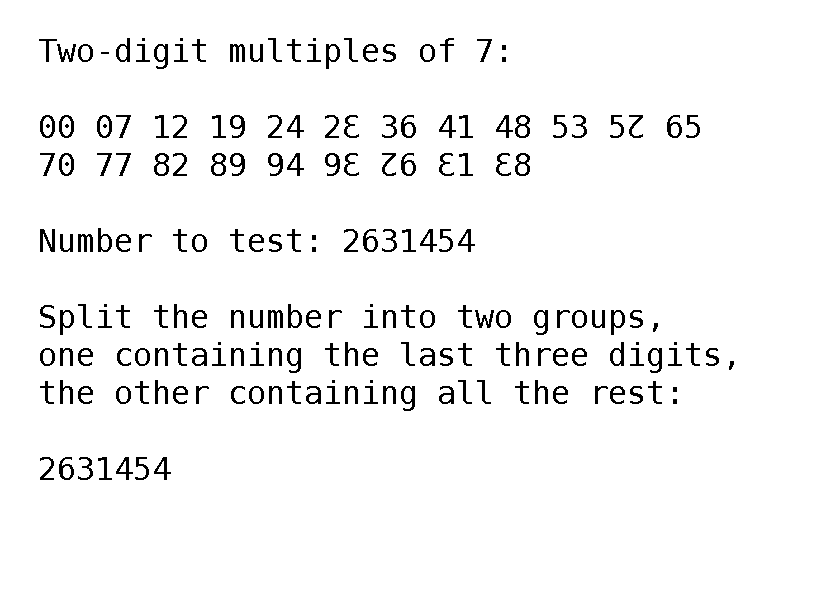Dozenal Society of America
Promoting base twelve and alternative base mathematics
Support the DSA
The Dozenal Society of America
The DSA is a voluntary, nonprofit education corporation, organized for the conduct of research and education of the public in the use of dozenal (also called duodecimal or base-twelve) in calculations, mathematics, weights and measures, and other branches of pure and applied science.

# Dozenal Divisibility Rules

The dozenal system, due to its many even divisors (it is the lowest abundant number), is particularly rich in divisibility tests; that is, it is often very easy to tell at a glance whether a given number is divisible by some particular integer. We are all familiar with the most basic of these divisibility tests; namely, whether a number is divisible by two, which is the same as asking whether it is even (divisible by two) or odd (not divisible by two). But dozenal offers easy divisibility tests for all integers lower than the dozen itself, with the sole exception of the prime number seven.

Integer Test
2 Number ends in 0, 2, 4, 6, 8, or X.
3 Number ends in 0, 3, 6, or 9.
4 Number ends in 0, 4, or 8.
5 Split, Promote, Discard (SPD). Split off the last two digits; add or subtract from them enough to make them divisible by five ("promote" them to a multiple of five); then discard them. Add or subtract the same amount from the remaining number as you did to the two digits you split off. Repeat until you have the last digit or two. If the last digit or two are zero or divisible by five, then the whole number is.*
6 Number ends in 0 or 6.
7 No reasonable test; there is a complicated one, the abbreviated cube-alpha test.**
8 • Second-to-last digit is even and last digit is 0 or 8.
• Second-to-last digit is odd and last digit is 4.
9 • Second-to-last digit is divisible by 3 and the last digit is 0 or 9.
• Second-to-last digit is one less than a multiple of 3 and the last digit is 3.
• Second-to-last digit is one more than a multiple of 3 and the last digit is 6.
X Divisible by both 2 and 5.
E Sum all the digits. If the sum is a multiple of E, then the number is divisible by eleven. Repeat by summing the digits of the sum if necessary.
10 Ends in 0.

These are merely the simplest tests for the integers less than or equal to the dozen itself; there are many others for many other integers. Treisaran, active on the DozensOnline forum, has composed a manual describing many of these, along with a much fuller description of the SPD test.

With these tests, we can easily determine whether a number is divisible by any of these numbers, often at a glance, and at the most with only a little thinking. Not merely an example of the richness of the dozenal system, divisibility tests are a powerful arithmetical tool.

* Treisaran has prepared an animated gif showing the workings of the SPD test, which makes it much simpler to grasp:** Treisaran has also prepared an animated gif showing the workings of the abbreviated power-alpha test, which makes it much easier to grasp (though not much easier to do):